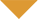# Calculating gearing on Bike FridaysSymptoms

I’m trying to calculate the gear-inches for my Pocket Rocket (451mm wheels). It has the SRAM 3×7 hub. Can you tell me:
1. What is the size of the wheel in inches?
2. What are the multipliers involved with the 3×7 hub?

Solutions

There are a variety of formulas to use, depending on the setup that you are using. The first piece of information you will need is the wheel size.

Rim Imperial Metric
406 18.4 in. 46.7cm
451 19.7 in. 50.0cm
349 16.1 in. 40.9cm

Evaluate the formulas below by using the above wheel sizes and the number of teeth on each of the cogs and chainrings. For bicycles without internal hubs:

((largest front chainring) x (wheelsize in inches)) 垨smallest cog) = high gear-inch

((smallest front chainring) x (wheelsize in inches)) 垨largest cog)= low gear-inch

For the SRAM 3×7 hub, you need to know the ratio that the hub provides, depending on what gear you are in. The ratios are as follows:

high = 1.36 middle = 1.00 low = 0.73

The formula for the interal hub bicycles is as follows:

(((front chainring) x (internal hub high gear)) x (wheelsize)) 垨smallest cog) = high gear-inch

(((front chainring) x (internal hub low gear)) x (wheelsize)) 垨largest cog) = low gear inch

This may seem confusing. There are resources on the Internet which may be of use. A gear calculator can be found at http://www.sheldonbrown.com/gears/.